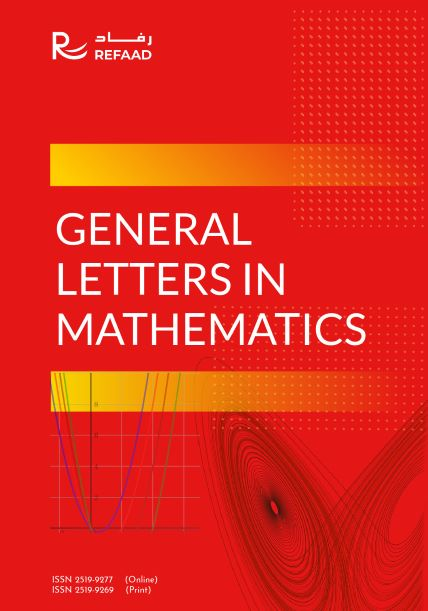#### General Letters in Mathematics (GLM) is devoted to publish high-quality papers dealing with original research on a wide range of mathematical subjects, including almost all areas of pure and applied mathematics. The journal also emphasizes articles dealing with statistics, mathematical aspects of computational theory, as well as applications of mathematics in many branches of science and engineering.

Scope:

• Algebra
• Calculus and analysis
• Geometry and topology
• Logic
• Number theory
• Applied harmonic analysis
• Approximation theory
• Asymptotic methods
• Bifurcation theory
• Coding theory
• Combinatorics
• Complex analysis
• Convex analysis
• Difference equations
• Differential equations
• Dynamical systems
• Fixed-Point theory
• Fourier analysis
• Fractals
• Fractional analysis
• Functional differential equations
• Fuzzy mathematics
• Geometry of banach spaces
• Scattering theory
• Semigroups of operators
• Signal theory
• Special functions
• Statistics
• Stochastic process
• Topology and applications
• Transport theory
• Ultrametric analysis
• Variational methods
• Integral equations
• Mathematical physics
• Matrix theory
• Number theory
• Numerical analysis
• Operator theory Optimal control
• Optimization wavelets
• Partial differential equations
• Probability
Current IssueBibliographic Information Email: editorglm@refaad.com DOI: 10.31559/GLM ISSN 2519-9277 (Online) ISSN 2519-9269 (Print) Freq. : Quarterly Language : English Articles : 165